Name: ___________________Date:___________________

 Email us to get an instant 20% discount on highly effective K-12 Math & English kwizNET Programs!

### High School Mathematics - 28.12 Construction of a Tangent to a Circle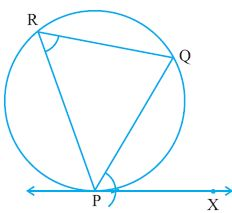Step 1: Draw any chord PQ of the circle through P as shown. Step 2: Take any point R on the major arc PQ. Join PR and QR. Step 3: COnstruct angle OPX equal to angle PRQ. PX is the required tangent at P. Steps to construct a tangent to a circle from an external point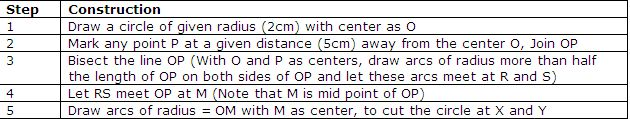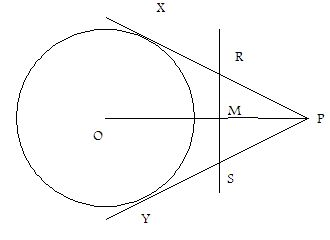Construction of Direct common tangents to 2 circles of equal radii whose centers are at a given distance from each other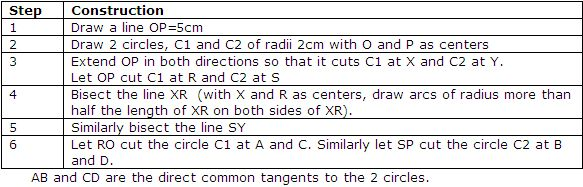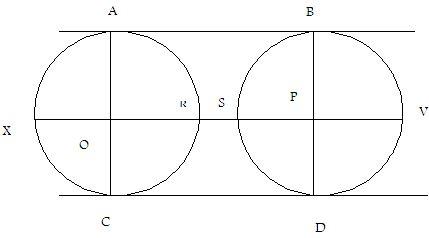Construction of Direct common tangents to 2 circles of different radii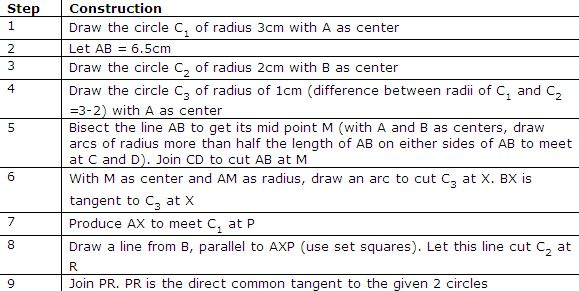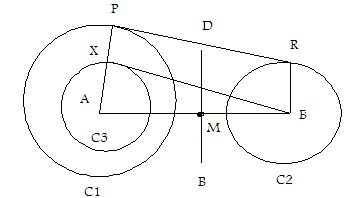Construction of tranverse tangents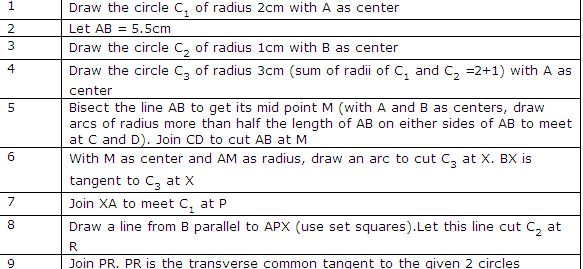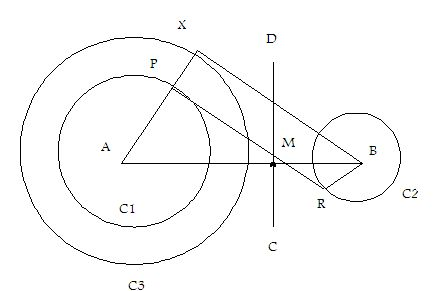Directions: Construct tangents to the following.
 Q 1: Draw direct common tangents to 2 circles of radii 6cm whose centers are 5cm apart. Answer: Q 2: Construct direct common tangents to 2 circles of radii 3cm and 2cm whose centers are 6.5cm apart.Answer: Q 3: Draw direct common tangents to 2 circles of radii 2cm whose centers are 5cm apart. Answer: Q 4: Answer: Q 5: Construct direct common tangents to 2 circles of radii 6cm and 4cm whose centers are 8.5cm apart.Answer: Q 6: Answer: Question 7: This question is available to subscribers only! Question 8: This question is available to subscribers only!